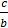### Sample Problem

Identify the slope and y-intercept of the following equation:

4x + 2y = 12

Slope:

y-intercept: (0, )

#### Solution

This equation is in standard form, ax + by = c.

The slope is, which is -2, and the y-intercept value is, which is 6.